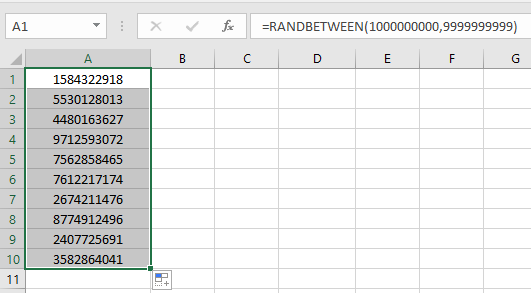# How to Generate Random Phone Numbers in Microsoft Excel 2010

In this article, we will learn to generate random list of phone numbers in Excel.

RANDBETWEEN function stands for Random number between the 2 numbers. To  generate a random phone number, follow the steps below.

RANDBETWEEN function returns random numbers between any two numbers.

Syntax:

=RANDBETWEEN ( bottom, top )

Bottom : The smaller of the 2 numbers.
Top : The larger of the 2 numbers.

As we know, a phone number has the following conditions:
1. It has 10 digits
So the smallest possible number comes out to be 1000000000 and the largest possible number comes out to be 9999999999

So use the formula shown below to generate phone numbers

Use the Formula:

= RANDBETWEEN ( 1000000000 , 9999999999 )

1000000000 : smaller one
9999999999 : larger oneJust enter the formula once and repeat results using the drag down option in excel as shown in the above snapshot.

As you can see, you have generated random phone numbers in seconds. You can use this formula as a random phone number generator.

Notes :

1. The function returns an error, if the first number argument ( bottom ) is larger than the second number argument ( top ).
2. The function returns error, if the argument to the function is non - numeric.

Hope it was explanatory enough and you know now how to generate phone numbers in Excel 2016. Find more articles on mathematical functions here. Please share your query below in the comment box. We will assist you.

Related Articles

Get Random number From Fixed Options

Get Random numbers between two numbers

Excel Random Selection: How to Get Random Sample From a Dataset

How to use the CHOOSE Function in Excel

How to use the RANDBETWEEN Function in Excel

Popular Articles

The VLOOKUP Function in Excel

Conditional Formatting with formula 2016

create Overlay Chart in Excel 2016

Terms and Conditions of use

The applications/code on this site are distributed as is and without warranties or liability. In no event shall the owner of the copyrights, or the authors of the applications/code be liable for any loss of profit, any problems or any damage resulting from the use or evaluation of the applications/code.